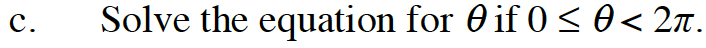### Home > PC3 > Chapter 8 > Lesson 8.3.3 > Problem8-117

8-117.
1. Yuka is trying to solve the following equation for θ, but is so confused by all the symbols; she does not know what to do. Homework Help ✎

2.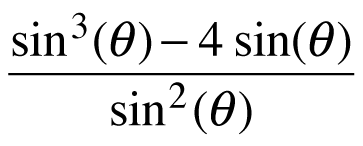= 3

1. Her friend Carlo suggests that she make the substitution u = sin(θ). Make this substitution and write the equation in terms of u.

2. Solve the equation for u.

3. Solve the equation for θ if 0 ≤ θ < 2π.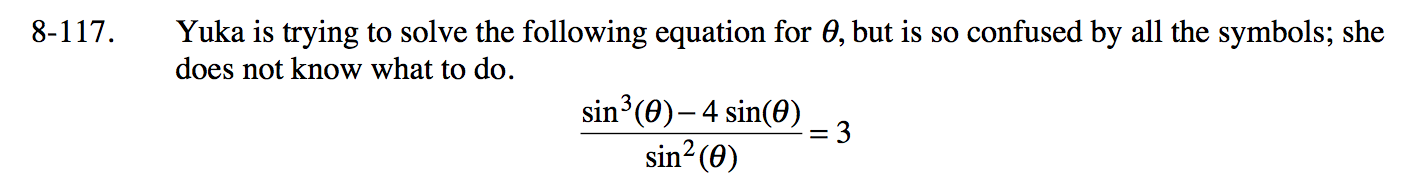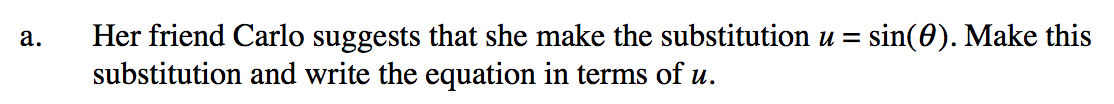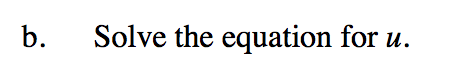Note: u ≠ 0 because you would have division by zero.
u2 − 4u = 3u2
u3 − 3u2 − 4u = 0
u(u2 − 3u − 4) = 0